# wthrmngr

Threshold settings manager

## Syntax

``thr = wthrmngr(opt,method,C,L)``
``thr = wthrmngr(opt,method,C,L,alpha)``
``thr = wthrmngr(opt,method,C,L,scale)``
``thr = wthrmngr(opt,method,swtdec,alpha)``
``thr = wthrmngr(opt,method,swtdec,scale)``
``thr = wthrmngr(opt,method,wpt)``
``thr = wthrmngr(opt,'rem_n0',X)``

## Description

`wthrmngr` returns a global threshold or level-dependent thresholds for wavelet-based denoising and compression. The function derives thresholds from the wavelet coefficients in a wavelet decomposition.

example

````thr = wthrmngr(opt,method,C,L)` returns the threshold for the `[C,L]` wavelet decomposition of the signal or image to compress or denoise. For signals, `[C,L]` is the output of `wavedec`. For images, `[C,L]` is the output of `wavedec2`.```

example

````thr = wthrmngr(opt,method,C,L,alpha)` returns the `[C,L]` wavelet decomposition threshold using the sparsity parameter `alpha`. For signals, `[C,L]` is the output of `wavedec`. For images, `[C,L]` is the output of `wavedec2`.To learn more about `alpha,` see `wdcbm` or `wdcbm2` for compression, and `wbmpen` for denoising.```
````thr = wthrmngr(opt,method,C,L,scale)` returns the `[C,L]` wavelet decomposition threshold using the type of multiplicative threshold rescaling specified in `scale`. For signals, `[C,L]` is the output of `wavedec`. For images, `[C,L]` is the output of `wavedec2`.The `'rigrsure'`, `'heursure'`, and `'minimaxi'` denoising methods are only applicable to signals.To learn more about multiplicative threshold rescaling, see `wden`.```
````thr = wthrmngr(opt,method,swtdec,alpha)` returns the level-dependent threshold for the stationary wavelet decomposition, `swtdec`, of the signal or image to denoise. `alpha` specifies the sparsity parameter (see `wbmpen`). For signals, `swtdec` is the output of `swt`. For images, `swtdec` is the output of `swt2`.Thresholds are derived from a subset of the coefficients in the stationary wavelet decomposition. For more information, see Coefficient Selection.```

example

````thr = wthrmngr(opt,method,swtdec,scale)` returns the level-dependent threshold for the stationary wavelet decomposition using the type of multiplicative threshold rescaling specified in `scale`. For signals, `swtdec` is the output of `swt`. For images, `swtdec` is the output of `swt2`.Thresholds are derived from a subset of the coefficients in the stationary wavelet decomposition. For more information, see Coefficient Selection.The `'rigrsure'`, `'heursure'`, and `'minimaxi'` denoising methods apply only to signals.To learn more about multiplicative threshold rescaling, see `wden`.```

example

````thr = wthrmngr(opt,method,wpt)` returns the global threshold for the wavelet packet decomposition, `wpt`, of the signal or image to compress or denoise.```
````thr = wthrmngr(opt,'rem_n0',X)` returns the global threshold to compress the signal or image, `X`, using the specified wavelet option and method `'rem_n0'`.If `opt` is `'dw1dcompGBL'` or `'dw2dcompGBL'`, thresholds are based on the finest-scale wavelet coefficients obtained using the Haar wavelet. If `opt` is `'wp1dcompGBL'` or `'wp2dcompGBL'`, thresholds are based on the finest-scale wavelet packet coefficients obtained using the Haar wavelet.```

## Examples

collapse all

Load and plot a noisy signal.

```load noisdopp plot(noisdopp) grid on title('Noisy Signal')```Generate a level 5 wavelet decomposition of the noisy signal using the order 4 Daubechies wavelet. Plot the coefficients.

```[c,l] = wavedec(noisdopp,5,'db4'); plot(c) grid on title('Wavelet Coefficients')```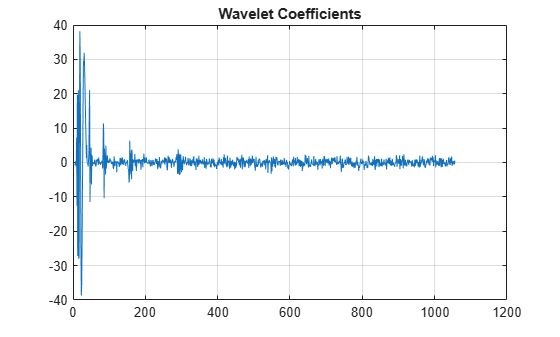Determine a global threshold for compressing the signal.

`thr = wthrmngr('dw1dcompGBL','bal_sn',c,l);`

The index of the first wavelet detail coefficient in `c` is `l(1)+1`. Apply the threshold to all the detail coefficients. Plot the thresholded coefficients. Observe that most of the coefficients have been set to 0.

```c(l(1)+1:end) = c(l(1)+1:end).*(c(l(1)+1:end)>thr); plot(c) grid on title('Thresholded Coefficients')```Reconstruct the signal from the thresholded coefficients. Plot the reconstruction.

```xrec = waverec(c,l,'db4'); plot(xrec) grid on title('Compressed Signal')```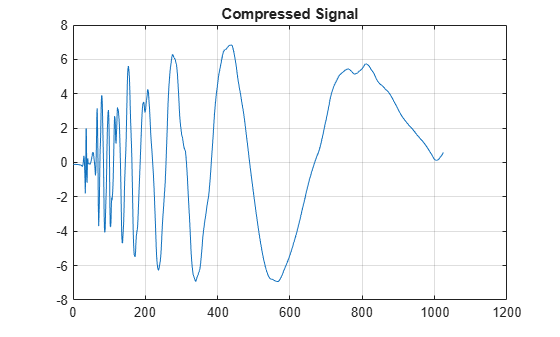Compress an image using the Birgé-Massart strategy.

Load an image and add white Gaussian noise. For purposes of reproducibility, set the random seed to the default value.

```rng default load sinsin x = X+18*randn(size(X));```

Obtain the 2-D discrete wavelet transform down to level 3 using the Daubechies least-asymmetric wavelet with 4 vanishing moments. Obtain the compression thresholds using the Birgé-Massart strategy with sparsity parameter, `alpha`, equal to 2.

```[C,L] = wavedec2(x,3,'sym4'); alpha = 2; THR = wthrmngr('dw2dcompLVL','scarcehi',C,L,alpha);```

Compress the image and display the result.

```xd = wdencmp('lvd',x,'sym4',3,THR,'s'); image(X) title('Original Image')``````figure image(x) title('Noisy Image')```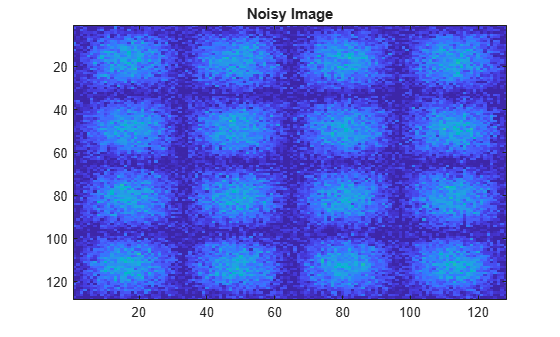```figure image(xd) title('Compressed Image')```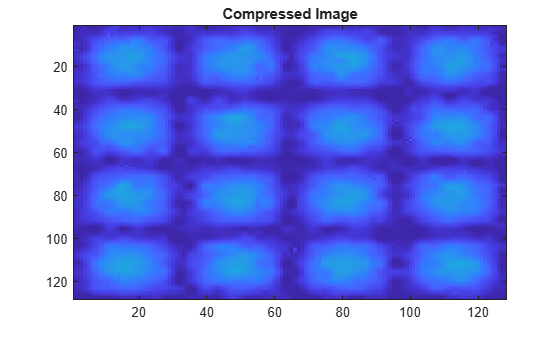This example uses a level-dependent threshold derived from the wavelet coefficients at each scale to implement hard thresholding with the stationary wavelet transform.

Load the noisy blocks signal. Obtain the stationary wavelet transform down to level 5 by using the Haar wavelet.

```load noisbloc L = 5; swc = swt(noisbloc,L,'haar');```

Make a copy of the wavelet transform coefficients. Determine the Donoho-Johnstone universal threshold based on the detail coefficients for each scale. Using the `'mln'` option, `wthrmngr` returns a 1-by-L vector, with every element equal to the universal threshold for the corresponding scale.

```swcnew = swc; ThreshML = wthrmngr('sw1ddenoLVL','sqtwolog',swc,'mln');```

Use the universal thresholds to implement hard thresholding. The thresholds are applied in a scale-dependent manner.

```for jj = 1:L swcnew(jj,:) = wthresh(swc(jj,:),'h',ThreshML(jj)); end```

Invert the stationary wavelet transform on the thresholded coefficients, `swcnew`. Plot the original signal and the denoised signal for comparison.

```noisbloc_denoised = iswt(swcnew,'haar'); plot(noisbloc) hold on plot(noisbloc_denoised,'r','linewidth',2) legend('Original','Denoised')```Denoise a noisy signal by applying a global threshold to a wavelet packet decomposition structure.

Load and plot a noisy signal.

```load noisdopp plot(noisdopp) grid on title('Noisy Signal')```Generate a level 3 wavelet packet decomposition of the noisy signal using the order 4 Daubechies wavelet.

`T = wpdec(noisdopp,3,'db4');`

Determine a global threshold for denoising the signal.

`thr = wthrmngr('wp1ddenoGBL','sqtwologuwn',T);`

Obtain the leaves from the wavelet packet decomposition tree `T` and apply the threshold to the leaves. Use hard thresholding.

```T1 = T; sorh = 'h'; cfs = read(T,'data'); cfs = wthresh(cfs,sorh,thr); T1 = write(T1,'data',cfs);```

Reconstruct the denoised signal from the thresholded coefficients. Plot the reconstruction.

```xrec = wprec(T1); plot(xrec) grid on title('Denoised Signal')```This example uses a level-independent threshold based on the finest-scale wavelet coefficients to implement hard thresholding with the stationary wavelet transform.

Load the noisy blocks signal. Obtain the stationary wavelet transform down to level 5 by using the Haar wavelet.

```load noisbloc L = 5; swc = swt(noisbloc,L,'haar');```

Make a copy of the wavelet transform coefficients. Determine the Donoho-Johnstone universal threshold based on the first-level detail coefficients. Using the `'sln'` option, `wthrmngr` returns a 1-by-L vector, with every element equal to the same value. Take the mean of the vector to obtain a scalar threshold.

```swcnew = swc; ThreshSL = mean(wthrmngr('sw1ddenoLVL','sqtwolog',swc,'sln'));```

Use the universal threshold to implement hard thresholding. The same threshold is applied to the wavelet coefficients at every level.

```for jj = 1:L swcnew(jj,:) = wthresh(swc(jj,:),'h',ThreshSL); end```

Invert the stationary wavelet transform on the thresholded coefficients, `swcnew`. Plot the original signal and the denoised signal for comparison.

```noisbloc_denoised = iswt(swcnew,'haar'); plot(noisbloc) hold on plot(noisbloc_denoised,'r','linewidth',2) legend('Original','Denoised')```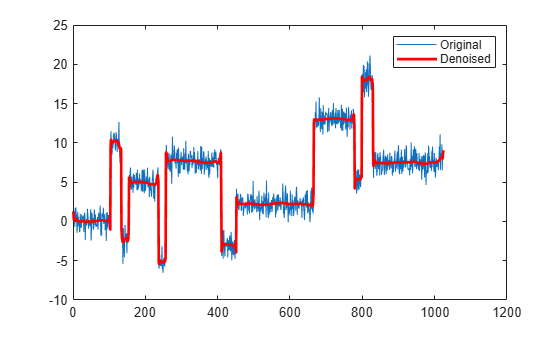## Input Arguments

collapse all

Type and dimension of compression or denoising, specified as one of the values listed in the tables that follow. `wthrmngr` returns thresholds appropriate for the option you specify.

With a discrete wavelet or wavelet packet decomposition of the data, you can compress or denoise that data. With a stationary wavelet decomposition of the data, you can only denoise the data.

For an explanation of which coefficients are used to determine the thresholds, see Coefficient Selection.

#### 1-D Discrete Wavelet Decomposition Options

In these options, `X` is the signal, the wavelet coefficients are in the vector `C`, and the lengths of the coefficient vectors are in `L`. The argument `alpha` is the sparsity parameter, and `scale` defines the multiplicative threshold rescaling.

For additional information regarding the wavelet decomposition, see `wavedec`. To learn more about `alpha` and `scale`, see `wdcbm` and `wden` respectively.

`opt`

Description

Valid Syntaxes
`'dw1dcompGBL'`

1-D compression using a global threshold

• ```thr = wthrmngr('dw1dcompGBL','rem_n0',X)```

• ```thr = wthrmngr('dw1dcompGBL','bal_sn',C,L)```

`'dw1dcompLVL'`

1-D compression using level-dependent thresholds

• ```thr = wthrmngr('dw1dcompLVL','scarcehi',C,L,alpha)```, where 2.5 < `alpha` < 10

• ```thr = wthrmngr('dw1dcompLVL','scarceme',C,L,alpha)```, where 1.5 < `alpha` < 2.5

• ```thr = wthrmngr('dw1dcompLVL','scarcelo',C,L,alpha)```, where 1 < `alpha` < 2

`'dw1ddenoLVL'`

1-D denoising using level-dependent thresholds

• ```thr = wthrmngr('dw1ddenoLVL','sqtwolog',C,L,scale)```

• ```thr = wthrmngr('dw1ddenoLVL','rigrsure',C,L,scale)```

• ```thr = wthrmngr('dw1ddenoLVL','heursure',C,L,scale)```

• ```thr = wthrmngr('dw1ddenoLVL','minimaxi',C,L,scale)```

• ```thr = wthrmngr('dw1ddenoLVL','penalhi',C,L,alpha)```, where 2.5 < `alpha` < 10

• ```thr = wthrmngr('dw1ddenoLVL','penalme',C,L,alpha)```, where 1.5 < `alpha` < 2.5

• ```thr = wthrmngr('dw1ddenoLVL','penallo',C,L,alpha)```, where 1 < `alpha` < 2

#### 2-D Discrete Wavelet Decomposition Options

In these options, `X` is the data, the wavelet coefficients are in the vector `C`, and the size of the coefficient matrices are in `L`. The argument `alpha` is the sparsity parameter, and `scale` defines the multiplicative threshold rescaling.

For additional information regarding the wavelet decomposition, see `wavedec2`. To learn more about `alpha` and `scale`, see `wdcbm2` and `wden` respectively.

`opt`

Description

Valid Syntaxes
`'dw2dcompGBL'`

2-D compression using a global threshold

• ```thr = wthrmngr('dw2dcompGBL','rem_n0',X)```

• ```thr = wthrmngr('dw2dcompGBL','bal_sn',C,L)```

• ```thr = wthrmngr('dw2dcompGBL','sqrtbal_sn',C,L)```

`'dw2dcompLVL'`

2-D compression using level-dependent thresholds

• ```thr = wthrmngr('dw2dcompLVL','scarcehi',C,L,alpha)```, where 2.5 < `alpha` < 10

• ```thr = wthrmngr('dw2dcompLVL','scarceme',C,L,alpha)```, where 1.5 < `alpha` < 2.5

• ```thr = wthrmngr('dw2dcompLVL','scarcelo',C,L,alpha)```, where 1 < `alpha` < 2

`'dw2ddenoLVL'`

2-D denoising using level-dependent thresholds

• ```thr = wthrmngr('dw2ddenoLVL','sqrtbal_sn',C,L)```

• ```thr = wthrmngr('dw2ddenoLVL','penalhi',C,L,alpha)```, where 2.5 < `alpha` < 10

• ```thr = wthrmngr('dw2ddenoLVL','penalme,C,L,alpha)```, where 1.5 < `alpha` < 2.5

• ```thr = wthrmngr('dw2ddenoLVL','penallo,C,L,alpha)```, where 1 < `alpha` < 2

• ```thr = wthrmngr('dw2ddenoLVL','sqtwolog',C,L,scale)```

#### 1-D Wavelet Packet Decomposition Options

In these options, `X` is the signal and `wpt` is the wavelet packet decomposition structure of the signal.

For additional information regarding the wavelet packet decomposition, see `wpdec`.

`opt`

Description

Valid Syntaxes
`'wp1dcompGBL'`

1-D compression using a global threshold

• ```thr = wthrmngr('wp1dcompGBL','rem_n0',X)```

• ```thr = wthrmngr('wp1dcompGBL','bal_sn',wpt)```

`'wp1ddenoGBL'`

1-D denoising using a global threshold

• ```thr = wthrmngr('wp1ddenoGBL','sqtwologuwn',wpt)```

• ```thr = wthrmngr('wp1ddenoGBL','sqtwologswn',wpt)```

• ```thr = wthrmngr('wp1ddenoGBL','bal_sn',wpt)```

• ```thr = wthrmngr('wp1ddenoGBL','penalhi',wpt)```

The `wpbmpen` function is used with the tuning parameter `ALPHA` = 6.25.

• ```thr = wthrmngr('wp1ddenoGBL','penalme',wpt)```

The `wpbmpen` function is used with the tuning parameter `ALPHA` = 2.

• ```thr = wthrmngr('wp1ddenoGBL','penallo',wpt)```

The `wpbmpen` function is used with the tuning parameter `ALPHA` = 1.5.

#### 2-D Wavelet Packet Decomposition Options

In these options, `X` is the data and `wpt` is the wavelet packet decomposition structure of the data.

For additional information regarding the wavelet packet decomposition, see `wpdec2`.

`opt`

Description

Valid Syntaxes
`'wp2dcompGBL'`

2-D compression using a global threshold

• ```thr = wthrmngr('wp2dcompGBL','rem_n0',X)```

• ```thr = wthrmngr('wp2dcompGBL','bal_sn',wpt)```

• ```thr = wthrmngr('wp2dcompGBL','sqrtbal_sn',wpt)```

`'wp2ddenoGBL'`

2-D denoising using a global threshold

• ```thr = wthrmngr('wp2ddenoGBL','sqtwologuwn',wpt)```

• ```thr = wthrmngr('wp2ddenoGBL','sqtwologswn',wpt)```

• ```thr = wthrmngr('wp2ddenoGBL','sqrtbal_sn',wpt)```

• ```thr = wthrmngr('wp2ddenoGBL','penalhi',wpt)```

The `wpbmpen` function is used with the tuning parameter `ALPHA` = 6.25.

• ```thr = wthrmngr('wp2ddenoGBL','penalme',wpt)```

The `wpbmpen` function is used with the tuning parameter `ALPHA` = 2.

• ```thr = wthrmngr('wp2ddenoGBL','penallo',wpt)```

The `wpbmpen` function is used with the tuning parameter `ALPHA` = 1.5.

#### 1-D Stationary Wavelet Decomposition Options

Denoising using level-dependent thresholds is the only option available for a 1-D stationary wavelet decomposition, `swtdec`. In this option, `alpha` is a sparsity parameter and `scale` defines the multiplicative threshold rescaling.

For more information regarding the stationary wavelet decomposition, see `swt`. To learn more about `alpha` and `scale`, see `wbmpen` and `wden` respectively.

`opt`Valid Syntaxes
`'sw1ddenoLVL'`
• ```thr = wthrmngr('sw1ddenoLVL','sqtwolog',swtdec,scale)```

• ```thr = wthrmngr('sw1ddenoLVL','rigrsure',swtdec,scale)```

• ```thr = wthrmngr('sw1ddenoLVL','heursure',swtdec,scale)```

• ```thr = wthrmngr('sw1ddenoLVL','minimaxi',swtdec,scale)```

• ```thr = wthrmngr('sw1ddenoLVL','penalhi',swtdec,alpha)```, where 2.5 < `alpha` < 10

• ```thr = wthrmngr('sw1ddenoLVL','penalme',swtdec,alpha)```, where 1.5 < `alpha` < 2.6

• ```thr = wthrmngr('sw1ddenoLVL','penallo',swtdec,alpha)```, where 1 < `alpha` < 2

Thresholds are based on a subset of the coefficients in the stationary wavelet decomposition. See Coefficient Selection for additional information.

#### 2-D Stationary Wavelet Decomposition Options

Denoising using level-dependent thresholds is the only option available for a 2-D stationary wavelet decomposition, `swtdec`. In this option, `alpha` is a sparsity parameter and `scale` defines the multiplicative threshold rescaling.

For more information regarding the stationary wavelet decomposition, see `swt2`. To learn more about `alpha` and `scale`, see `wbmpen` and `wden` respectively.

`opt`Valid Syntaxes
`'sw2ddenoLVL'`
• ```thr = wthrmngr('sw2ddenoLVL','sqrtbal_sn',swtdec)```

• ```thr = wthrmngr('sw2ddenoLVL','penalhi',swtdec,alpha)``` where 2.5 < `alpha` < 10

• ```thr = wthrmngr('sw2ddenoLVL','penalme',swtdec,alpha)``` where 1.5 < `alpha` < 2.5

• ```thr = wthrmngr('sw2ddenoLVL','penallo',swtdec,alpha)``` where 1 < `alpha` < 2

• ```thr = wthrmngr('sw2ddenoLVL','sqtwolog',swtdec,scale)```

Thresholds are based on a subset of the coefficients in the stationary wavelet decomposition. See Coefficient Selection for additional information.

Thresholding method, specified as one of the values listed here.

`method`Description
`'scarcehi'`Uses Birgé-Massart strategy for determining thresholds.
`'scarceme'`Uses Birgé-Massart strategy for determining thresholds.
`'scarcelo'`Uses Birgé-Massart strategy for determining thresholds.
`'sqtwolog'`Uses fixed-form universal threshold. See `'sqtwolog'` option in `wden`.
`'sqtwologuwn'`Uses fixed-form universal threshold. See `'sqtwolog'` option in `wden` when used with '`sln`' option.
`'sqtwologswn'`Uses fixed-form universal threshold. See `'sqtwolog'` option in `wden` when used with `'mln'` option.
`'rigsure'`Uses soft threshold estimator rule based on Stein's Unbiased Estimate of Risk. See `'SURE'` option in `wdenoise`.
`'heursure'`Uses mixture of `'rigsure'` and `'sqtwolog'`. See `'heursure'` option in `wden`.
`'minimaxi'` Uses a fixed threshold chosen which yields minimax performance. See `'Minimax'` option in `wdenoise`.
`'penalhi'`Used to define Birgé-Massart strategy for determining thresholds.
`'penalme'`Used to define Birgé-Massart strategy for determining thresholds.
`'penallo'`Used to define Birgé-Massart strategy for determining thresholds.
`'rem_n0'`Returns a threshold close to 0. A typical `THR` value is `median(abs(coefficients))`.
`'bal_sn'`Returns a threshold such that the percentages of retained energy and number of zeros are the same.
`'sqrtbal_sn'`Returns a threshold equal to the square root of the value such that the percentages of retained energy and number of zeros are the same.

Data Types: `char`

Input data, specified as a real-valued vector or real-valued matrix.

Data Types: `double`

Wavelet expansion coefficients of the data to be compressed or denoised, specified as a real-valued vector. If the data is one-dimensional, `C` is the output of `wavedec`. If the data is two-dimensional, `C` is the output of `wavedec2`.

Example: ```[C,L] = wavedec(randn(1,1024),3,'db4')```

Data Types: `double`

Size of wavelet expansion coefficients of the signal or image to be compressed or denoised, specified as a vector or matrix of positive integers.

For signals, `L` is the output of `wavedec`. For images, `L` is the output of `wavedec2`.

Example: ```[C,L] = wavedec(randn(1,1024),3,'db4')```

Data Types: `double`

Sparsity parameter used for compressing or denoising data, specified as a positive scalar greater than 1 and less than 10. See `wdcbm`, `wdcbm2`, and `wbmpen` for additional information.

Data Types: `double`

Multiplicative threshold rescaling, specified as one of the following:

• `'one'` — No rescaling

• `'sln'` — Rescaling using a single estimation of level noise based on first-level coefficients

• `'mln'` — Rescaling using a level-dependent estimation of level noise

For more information, see `wden`.

Stationary wavelet decomposition structure of data to be compressed or denoised, specified as a real-valued matrix. If the data is one-dimensional, `swtdec` is the output of `swt`. If the data is two-dimensional, `swtdec` is the output of `swt2`.

Example: ```swtdec = swt2(randn(256),3,'db1')```

Data Types: `double`

Wavelet packet decomposition structure of the data to be compressed or denoised. If the data is one-dimensional, `wpt` is the output of `wpdec`. If the data is two-dimensional, `wpt` is the output of `wpdec2`.

Example: ```wpt = wpdec(randn(1,1024),5,'db1')```

## Output Arguments

collapse all

Threshold, returned as a real-valued scalar for global thresholds, or a real-valued vector or matrix for level-dependent thresholds.

Data Types: `double`

## Tips

• To denoise 1-D signals, consider using the Wavelet Signal Denoiser. The app visualizes and denoises real-valued 1-D signals using default parameters. You can also compare results. In addition, you can also recreate the denoised signal in your workspace by generating a MATLAB® script, which uses the `wdenoise` function.

## Algorithms

collapse all

### Coefficient Selection

A critically sampled wavelet or wavelet packet decomposition involves decimating coefficients by a factor of 2 at each stage of the decomposition. Decimation does not occur in the nondecimated stationary wavelet decomposition.

`wthrmngr` derives denoising and compression thresholds from the wavelet coefficients. For a critically sampled wavelet or wavelet packet decomposition, the option and method determine whether all wavelet coefficients or only the finest scale coefficients are used.

For the stationary wavelet decomposition, `wthrmngr` always uses a subset of the wavelet coefficients. When computing the denoising thresholds of an `N`-level stationary wavelet decomposition, the algorithm first subsamples the wavelet coefficients at level `k` by a factor of `2k`, for ```k = 1,…,N```. The algorithm uses this subset of coefficients to determine the thresholds. Most of the coefficients in the stationary wavelet decomposition are not considered.

### Birgé-Massart Strategy

The Birgé-Massart strategy for determining thresholds depends on several different parameters. You specify the wavelet decomposition and a thresholding method. You can also specify a sparsity parameter, `alpha`, or a specific multiplicative threshold rescaling, `scale`. Based on your inputs, `wthrmngr` derives the necessary Birgé-Massart parameters. The parameters depend on the dimension of the signal, and the total number, `N`, of coefficients at the coarsest scale of wavelet decomposition.

If the thresholding method is `'scarcehi'`, `'scarceme'`, or `'scarcelo'`, the `wthrmngr` executes either `wdcbm` or `wdcbm2`. If the thresholding method is `'penalhi'`, `'penalme'`, or `'penallo'`, then `wthrmngr` executes either `wbmpen` or `wpbmpen`.

Thresholding Method

Description

`'scarcehi'`
• If the signal is 1-D, then `wdcbm` is used with input argument `M` = `N`.

• If the signal is 2-D, then `wdcbm2` is used with `M` = `4*N`.

`'scarceme'`
• If the signal is 1-D, then `wdcbm` is used with input argument `M` = `3*N/2`.

• If the signal is 2-D, then `wdcbm2` is used with input argument with `M` = `16*N/3`.

`'scarcelo'`
• If the signal is 1-D, then `wdcbm` is used with input argument `M` = ```2 N```.

• If the signal is 2-D, then `wdcbm2` is used with input argument `M` = `32*N/3`.

`'penalhi'`
• If the input is a wavelet decomposition, then `wbmpen` is used with `ALPHA` = `5*(3*alpha+1)/8`.

• If the input is a wavelet packet decomposition, then `wpbmpen` is used `ALPHA` = `6.25`.

`'penalme'`
• If the input is a wavelet decomposition, then `wbmpen` is used with `ALPHA` = `(alpha+5)/8`.

• If the input is a wavelet packet decomposition, then `wpbmpen` is used `ALPHA` = `2`.

`'penallo'`
• If the input is a wavelet decomposition, then `wbmpen` is used with `ALPHA` = `(alpha+3)/4`.

• If the input is a wavelet packet decomposition, then `wpbmpen` is used `ALPHA` = `1.5`.

 Birgé, L., and P. Massart. “From Model Selection to Adaptive Estimation.” Festschrift for Lucien Le Cam: Research Papers in Probability and Statistics (E. Torgersen, D. Pollard, and G. Yang, eds.). New York: Springer-Verlag, 1997, pp. 55–88.